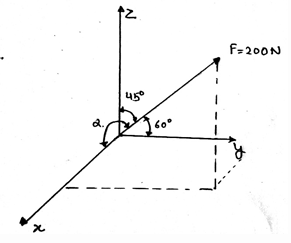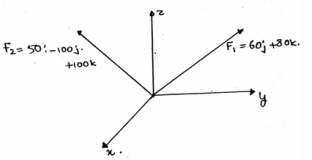Courses

# Test: Cables Subjected To A Distributed Load

## 15 Questions MCQ Test Engineering Mechanics | Test: Cables Subjected To A Distributed Load

Description
This mock test of Test: Cables Subjected To A Distributed Load for Mechanical Engineering helps you for every Mechanical Engineering entrance exam. This contains 15 Multiple Choice Questions for Mechanical Engineering Test: Cables Subjected To A Distributed Load (mcq) to study with solutions a complete question bank. The solved questions answers in this Test: Cables Subjected To A Distributed Load quiz give you a good mix of easy questions and tough questions. Mechanical Engineering students definitely take this Test: Cables Subjected To A Distributed Load exercise for a better result in the exam. You can find other Test: Cables Subjected To A Distributed Load extra questions, long questions & short questions for Mechanical Engineering on EduRev as well by searching above.
QUESTION: 1

### In the support system of the bridges and trolley wheel ____________ form the main loading carrying element in the structure.

Solution:

The cable is a support system which is used to transfer the loadings in the different structures. The main motto is to make the structure stable. This is probably done with the help of making the distribution of the load which is being given to the structures equally by the help of the cables.

QUESTION: 2

### What is the dot product of the components of the force vector shown in the figure, i.e. the dot product of the z-axis component and the y-axis component of the force?Solution:

The dot product of any two vectors is having the cosine function in it. It is being multiplied by product of the magnitudes of the vectors. So if the angle is 90°, then the value of cosine function would be zero, thus 0. And the value of the sine angle is 1, thus the result.

QUESTION: 3

### The assumptions for the calculations for cables under distributed load are done for the cables. In that one of the assumption is that the cable is ___________

Solution:

The assumptions are done so as to make the calculations easy. Though the assumptions make the small errors to not count over the big dat. But still if the assumptions are made then the calculations are easy. Thus the assumption taken as the cable is perfectly flexible.

QUESTION: 4

The force on the cables under distributed load is not neglected in the calculations of the load distribution.

Solution:

The cable is a support system which is used to transfer the loadings in the different structures. The main motto is to make the structure stable. In calculation the self-weight of the cable is neglected and the load is calculated. The forces are acting in the vertically downward direction.

QUESTION: 5

The assumptions for the calculations are done for the cables under distributed load. In that one of the assumption is that the cable is flexible and the other is that the cable is ___________

Solution:

The assumptions are done so as to make the calculations easy. Though the assumptions make the small errors to not count over the big dat. But still if the assumptions are made then the calculations are easy. Thus the assumption taken as the cable is inextensible.

QUESTION: 6

The various points in the cables under distributed load is facing a ____________ tensile force.

Solution:

The various points in the cables are facing constant forces. The forces are tensile forces. This is because as the cables are subjected to loadings the loads tends to stretch the cables in the direction parallel to its round area region. Thus the points face tensile forces which are parallel to the cable length.

QUESTION: 7

Due to which property the cables under distributed load, it offers no resistance to bending?

Solution:

Due to the flexibility property the cable, it offers no resistance to bending. As the bending is seen in the beams and all the solid structures. Thus the bending moment produced in the cables are not affecting the cables much. Thus no affect by bending, i.e. no resistance to bending.

QUESTION: 8

Flexible cables under distributed load with chains combine ___________ with lightness and often are used in structures for support.

Solution:

The chains with the cables are used to support the structures. They ae there for the transfer of the loading from one point to another point. This means that the cables not only provides strength to the structure but also sufficiently transfers the loads that are being added to it.

QUESTION: 9

The tensile force acting on the cables under distributed load is in which direction w.r.t the cable?

Solution:

Due to the flexibility property the cable, it offers no resistance to bending. As the bending is seen in the beams and all the solid structures. Thus the bending moment produced in the cables are not affecting the cables much. So the tensile force which is being produced is acting in the tangential direction to the points of the cable along its lengths.[/expand]

QUESTION: 10

If the unknown variables in the calculations for cables under distributed load are more than the known quantities, then the number of equations required to solve all the unknown variables are?

Solution:

Whatever be the calculation involved, the unknown variables will be ultimately come out from the equations. The proper use of the known quantities and the multiuse of various calculation techniques will ultimately give up the results. Thus whether it may be the case of the cables or the beams the equations if applied properly will result in the determination of the unknowns.

QUESTION: 11

Solution:

The cable takes the shape of straight line when subjected to the loadings. As the loadings are straight, acting vertically downwards, they stretch the cables and then make them come in the shape of the straight line. Thus the loadings make the cables come in the straight line.

QUESTION: 12

The cables under distributed load weight become significant in the calculations of the loadings when the cables are used in the transmission lines and guys for radio antennas.

Solution:

The cable is a support system which is used to transfer the loadings in the different structures. The main motto is to make the structure stable. But in the calculations of the loadings when the cables are used in the transmission lines and guys for antennas the self-weight of the cable is not neglected and the load is calculated.

QUESTION: 13

Solution:

The loading in the cables doesn’t affect the geometry of the cables. This is because of the assumptions which we have taken. The first one that the cables are perfectly elastic. And the second one that the cables are inextensible.

QUESTION: 14

What is the magnitude of the resultant vector’s unit vector? (Resultant vector of the two vectors shown in the figure below)Solution:

The question asked is asking for the magnitude of the unit vector of the resultant vector, thus the answer is 1. Whether the magnitude of the vector be any quantity, but the unit vector will have the magnitude equal to unity. And the direction given by the unit vector will be in the same direction as that of the vector.

QUESTION: 15

Being inextensible the cables under distributed load has ___________ length.

Solution:

The assumption that the cables are inextensible the main fact that comes out is that the cable length is constant throughout. Thus in the calculations also the fact that the length is constant makes a huge time saving tool. Thus the length of the cables are to be assumed constant if the assumption is taken as they are inextensible.